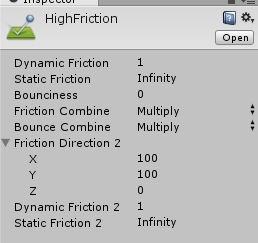# Physics behaves more strangely with Time? Bouncing and Sliding Objects

Is there any plausible reason why Physics would act more and more strangely over time?

I have gameobjects that spawn and destroy over time.

As time progresses the way these objects react to Colliders becomes more and more strange. There is no Time factor in my collision scripts.

Things that are affected most are Bounce (increasing) and Friction (decreasing).

The number of gameobjects doesn’t seem to be an issue IN THIS CASE as having few or many gameObjects early on will not behave this way but having few or many later on will.

Is my FixedUpdate() dropping steps? I’m getting a stable 80 fps!

EDIT: Ive added a HIGH FRICTION Physic Material and it has NO EFFECT on this issue!If you want to just rotate the gravity about the device Z axis (perpendicular to the screen), zero the Z axis and normalize the accelerometer value, then multiply it by gravityVal :

``````function Update ()
{
dir = Input.acceleration;
dir.x = -dir.x; // mirror X as you were already doing
dir.z = 0; // ignore Z axis
dir.Normalize();
Physics.gravity = gravityVal * dir.normalized;
}
``````

If the accelerometer is too jerky, filter it with a “Lerp filter”:

``````...
public smoothSpeed: float = 5;

function Update ()
{
var temp: Vector3 = Input.acceleration;
temp.x = -temp.x; // mirror X as you were already doing
temp.z = 0; // ignore Z axis
temp.Normalize(); // normalize it
dir = Vector3.Lerp(dir, temp, smoothSpeed * Time.deltaTime);
Physics.gravity = gravityVal * dir;
}
``````

I actually hacked it.

Solved with my other Q/A